July 17, 2019

# Introduction

• Chapter 23 of Introduction to Algorithms (3rd Edition), Thomas H. Cormen, Charles E. Leiserson, Ronald L. Rivest, and Clifford Stein.
• Minimum Spanning Trees have many real-world applications

# Wiring Electronic Circuits Problem (1)

• Design of electronic circuits
• Interconnect pins of components, pins are electrically equivalent
• Interconnect $$n$$ pins to $$n-1$$ cables (each to a pair of pins)
• Prefer wiring using least amount of wire
• Model problem with an undirected graph
• $$V$$, set of pins
• $$E$$, set of conections between pins
• $$W$$, cost of connecting two pins associated to the edges

# Wiring Electronic Circuits Problem (2)

• Find the acyclic subset $$T \subseteq E$$ connecting all vertices with the minimum weight
$w(T) = \sum_{(u,v) \in T} w(u,v)$
• $$T$$ is acyclic and connects all vertices
• $$T$$ is known as a spanning tree
• The problem of finding this tree is known as the minimum spanning tree problem

# Algorithms to Cover

• Kruskal
• Prim
• Execution time
• $$O(E \lg V)$$, implemented with binary heaps
• Enhancement for Prim
• $$O(E + V \lg V)$$ with Fibonacci heaps
• Enhancement when $$|V|$$ is much smaller than $$|E|$$
• Both algorithms are greedy
• Do not usually guarantee finding global optimal solutions
• For $$MST$$ can be proved that some strategies do find an $$ST$$ with minimum weight

# Growing an $$MST$$

• Given an undirected connected graph, $$G = (V, E)$$ with a weights function $$w : E \rightarrow R$$
• We want to find an $$MST$$ for $$G$$
• We could consider a greedy strategy
• Generic algorithm
•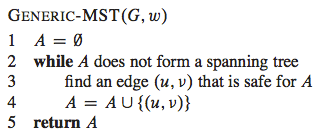# Loop Invariant - Generic Algorithm - $$MST$$

• Before each iteration
• $$A$$ is a subset of an $$MST$$
• Each step
• Determine an edge $$(u, v)$$ that can be added to $$A$$ without violating this invariant
• $$A \cup \{(u, v)\}$$ is also a subset of the $$MST$$
• An edge with this characteristic is called a safe edge for $$A$$, because we can add it in a safe way, keeping the invariant

# Generic MST Algorithm

• Initialization
• After line 1, set $$A$$ trivially satisfies the loop invariant
• Maintenance
• The loop in lines 2 - 4 keeps the invariant by adding only safe edges
• Termination
• All edges added to $$A$$ are in the $$MST$$, then set $$A$$ returned in line 5 should be an $$MST$$# How Can We Find Safe Edges?

• This is the hard part of the process
• Rule to recognize safe edges
• Theorem 23.1

# Theorem 23.1

• Let $$G = (V, E)$$ be a connected, undirected graph with a real-valued weight function $$w$$ defined on $$E$$. Let $$A$$ be a subset of $$E$$ that is included in some minimum spanning tree for $$G$$, let $$(S, V - S)$$ be any cut of $$G$$ that respects $$A$$, and let $$(u, v)$$ be a light edge crossing $$(S, V - S)$$. Then, edge $$(u, v)$$ is safe for $$A$$.

# Definitions

• A CUT $$(S, V - S)$$ of an undirected graph $$G = (V, E)$$ is a partition of $$V$$
• An edge $$(u, v) \in E$$ CROSSES cut $$(S, V - S)$$ if any of its endpoints is in $$S$$ and the otherone is in $$V - S$$
• We say that a cut RESPECTS a set $$A$$ of edges if no edge in $$A$$ crosses the cut.
• An edge is a LIGHT EDGE crossing a cut if its weight is the minimum of the edges crossing the cut
• An edge is a light edge satisfying a property if its weight is the minimum among any edge satisfying the property

# $$MST$$ for a Connected Graph

• Total weight: 37
• It isn’t unique, changing $$(b, c)$$ for $$(a, h)$$ we have another $$MST$$ with weight 37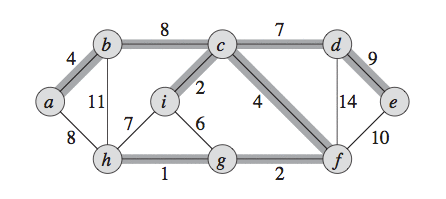# Examples of a Cut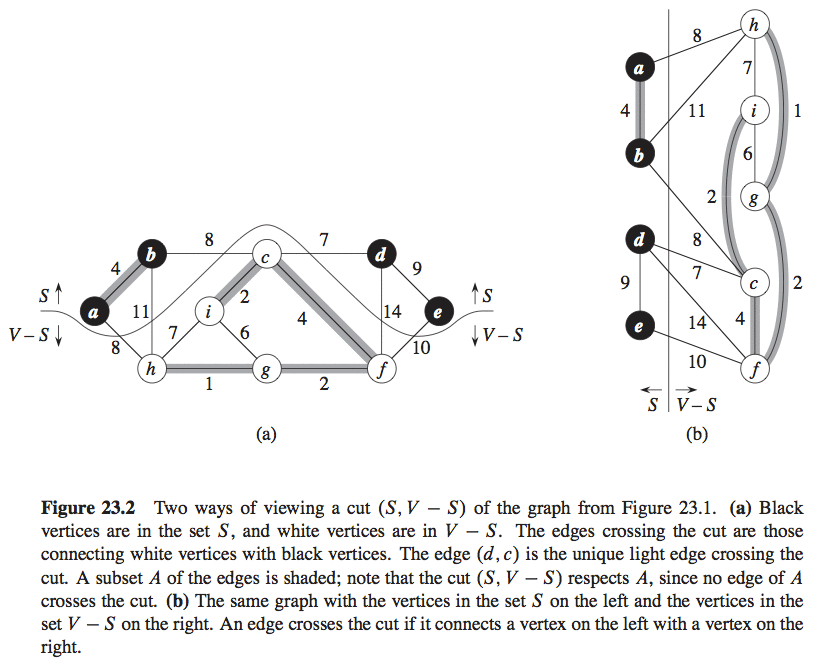# Theorem 23.1, to Recognize Safe Edges

• Given $$G = (V, E)$$ a connected and undirected graph with a weights function $$w$$ with real values defined over $$E$$
• Let $$A$$ be a subset of $$E$$ included in an $$MST$$ of $$G$$, and let $$(S, V - S)$$ be any cut of $$G$$ that respects $$A$$, and let $$(u, v)$$ be a light edge crossing $$(S, V - S)$$. Then, edge $$(u, v)$$ is safe for $$A$$

# Proof of Theorem 23.1

• Let $$T$$ be an $$MST$$ that includes $$A$$
• We assume that $$T$$ doesn’t contain light edge $$(u, v)$$
• We will build another $$MST$$, $$T^{\prime}$$ that includes $$A \cup \{(u, v)\}$$
• Cut and paste technique
• Proving that $$(u, v)$$ is a safe edge for $$A$$

# Proof of Theorem 23.1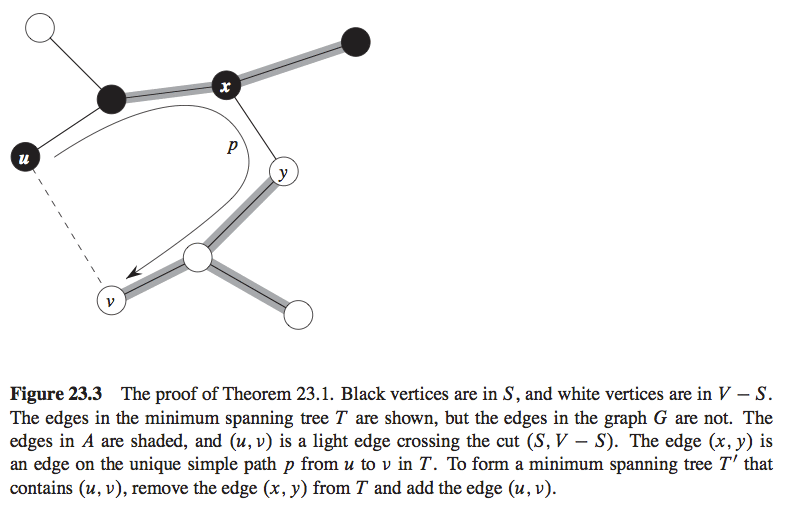# Proof of Theorem 23.1

• The edge $$(u, v)$$ forms a cycle with the edges in the path $$p$$ from $$u$$ to $$v$$ in $$T$$
• Because $$u$$ and $$v$$ are in opposite sides of the cut $$(S, V - S)$$, then there is at least an edge in $$T$$ in the path $$p$$ that also crosses the cut
• Let $$(x, y)$$ be that edge that also crosses the cut
• $$(x, y)$$ is not in $$A$$ because the cut respects $$A$$
• Because $$(x, y)$$ is in the unique path from $$u$$ to $$v$$ in $$T$$, if we remove $$(x, y)$$ we break $$T$$ in two components
• Adding $$(u, v)$$ we reconnect the components and form a new $$MST$$, $$T^{\prime} = T - \{(x, y)\} \cup \{(u, v)\}$$

# Proof of Theorem 23.1

• We now prove that $$T^{\prime}$$ is also an $$MST$$
• Because $$(u, v)$$ is a light edge that crosses $$(S, V - S)$$ and $$(x, y)$$ also crooses this cut
• $$w(u, v) \leq w(x, y)$$
• Then
• $$w(T^{\prime}) = w(T) - w(x, y) + w(u, v) \leq w(T)$$
• But $$T$$ is an $$MST$$, then $$w(T) \leq w(T^{\prime})$$
• Then $$T^{\prime}$$ must also be an $$MST$$

# Proof of Theorem 23.1

• Now we prove that $$(u, v)$$ is really a safe edge for $$A$$
• As $$A \subseteq T^{\prime}$$ because $$A \subseteq T$$ and $$(x, y) \notin A$$
• Then $$A \cup \{(u, v)\} \subseteq T^{\prime}$$
• Consequently, as $$T^{\prime}$$ is an $$MST$$, $$(u, v)$$ is safe for $$A$$

# Corolary 23.2

• Let $$G = (V, E)$$ be a connected and undirected graph with a weights function $$w$$ defined over $$E$$ with real values
• Let $$A$$ be a subset of $$E$$ that is included in some $$MST$$ of $$G$$
• Let $$C = (V_C, E_C)$$ a connected component (tree) in the forest $$G_A =(V, A)$$
• If $$(u, v)$$ is a light edge that connects $$C$$ to any other component in $$G_A$$, then $$(u, v)$$ is safe for $$A$$

# Kruskal’s and Prim’s Algorithms

• Variants of the generic algorithm
• Use a specific rule to determine a safe edge
• Kruskal
• Set $$A$$ is a forest
• Safe edge added $$A$$ is always the edge with the lowest weight in the graph that connects two distinct components
• Prim’s
• Set $$A$$ forms a unique tree
• The safe edge added to $$A$$ is always the edge with the lowest weight that connects the tree to a vertex that is not in the tree

# Kruskal

• The safe edge to add to the forest is that edge $$(u, v)$$ that connects any of two trees in the forest but with the lowest weight
• Because $$(u, v)$$ must be a light edge that connects $$C_1$$ to other tree
• Corolary 23.2 implies that $$(u, v)$$ is a safe edge for $$C_1$$
• Kruskal’s is greedy

# Kruskal’s Algorithm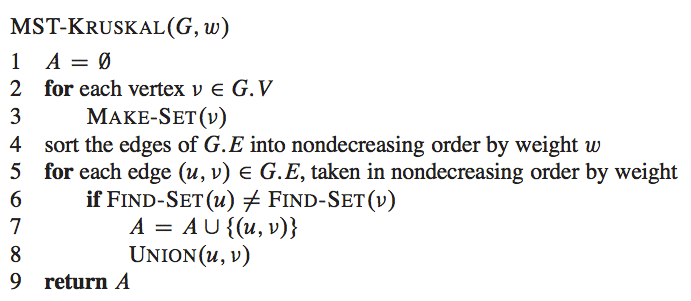# Kruskal’s Example (1)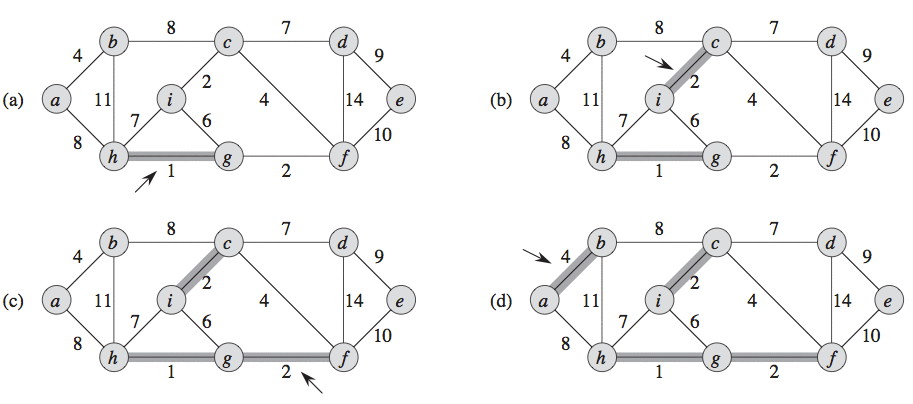# Kruskal’s Example (2)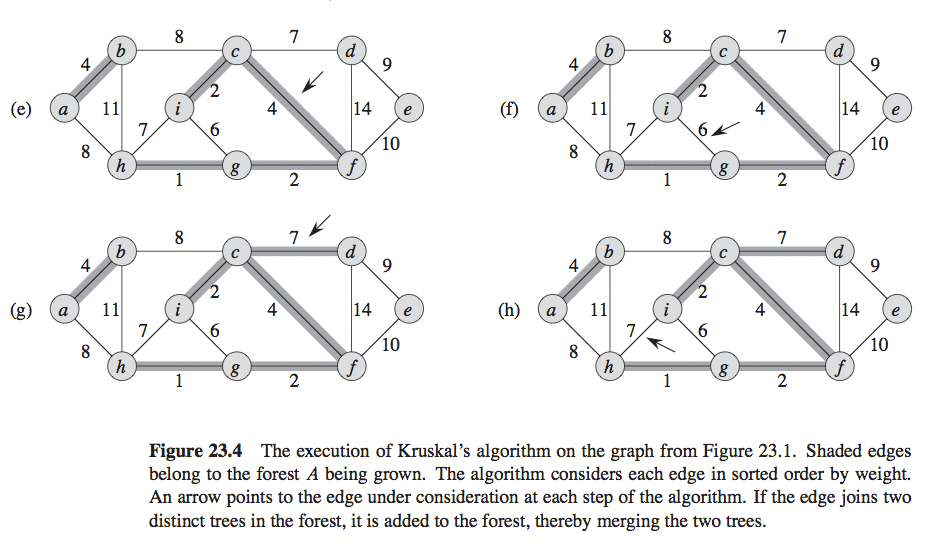# Kruskal’s Example (3)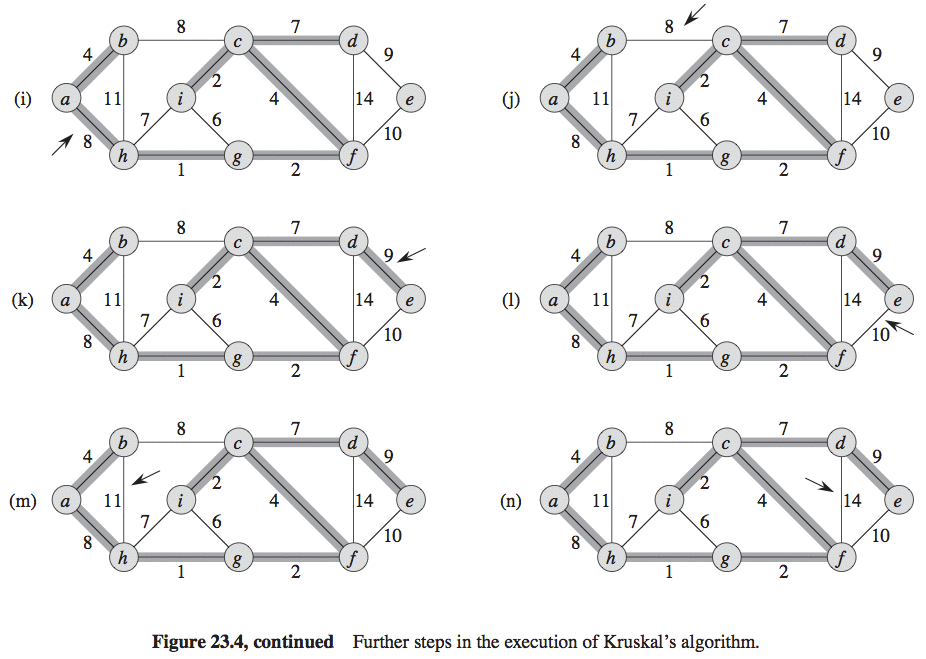# Kruskal’s Execution Time

• It depends on the implementation of the disjoint-set data structure
• $$O(ElgV)$$

# Prim’s

• Similar to the Dijkstra algorithm to find shortest paths in a graph (we will see it as well)
• Property
• Edges in set $$A$$ always form a tree
• The tree is initialized with an arbitrary root vertex and it is grown until the tree is expanded to all vertices in $$V$$
• Each step a light edge is added to the tree $$A$$ that connects it with a vertex that hasn’t been connected
• According to corolary 23.2, the rule adds only edges that are safe for $$A$$, then, at the end, the edges of $$A$$ form an $$MST$$
• Prim’s is greedy

# Prim’s Algorithm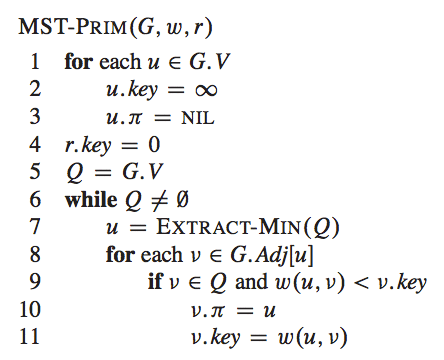# Prim’s Algorithm

• Priority Queue on a key field
• For each vertex $$V$$, $$key[v]$$ is the minimum weight for any edge that connects $$v$$ to an edge in the tree
• By convention $$key[v] = \infty$$ if that edge doesn’t exist
• $$\pi[v]$$ names the father of $$v$$ in the tree

# Homework

• Study the loop invariant of the $$MST-PRIM$$ algorithm

• Show that a graph has a unique minimum spanning tree if, for every cut of the graph, there is a unique light edge crossing the cut.

• What happens if we have negative weights in a graph while constructing a MST?

# Prim’s Example (1)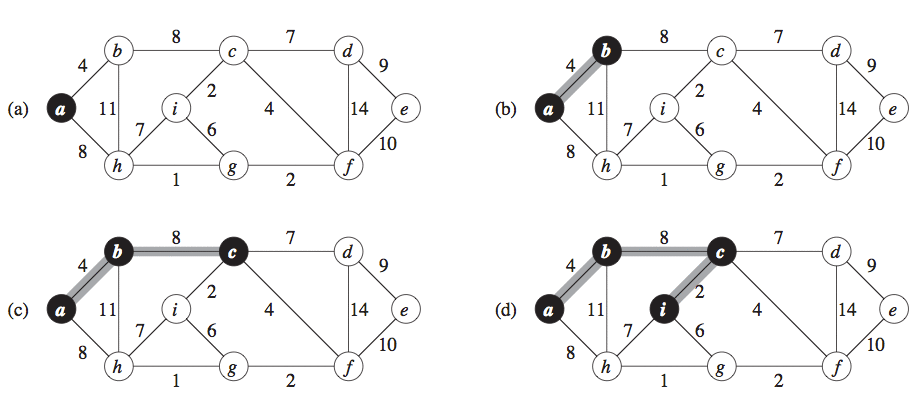# Prim’s Example (2)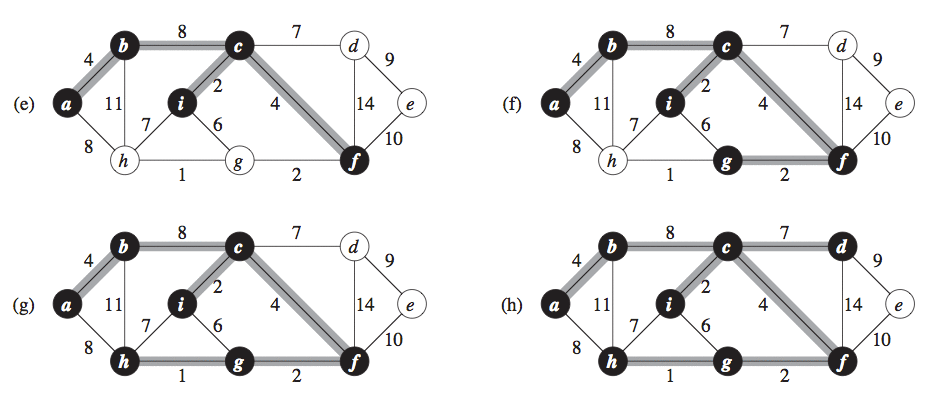# Prim’s Example (3)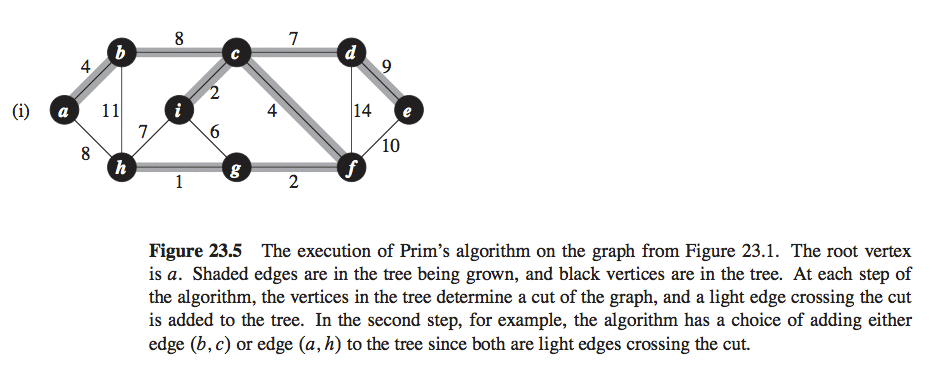# Prim’s Execution Time

• $$O(ElgV)$$
• It can be enhanced with Fibonacci-Heaps
• $$O(E + VlgV)$$

# Exercise

• We need to find the lowest cost for the road network connecting 5 cities. The cities and the cost to build the road between them are:
• Cities: a, b, c, d, e
• a-b: 3
• a-c: 5
• a-d: 11
• a-e: 9
• b-c: 3
• b-d: 9
• b-e: 8
• c-e: 10
• d-e: 7
• Find the lowest cost road using the Kruskal’s and Prim’s algorithms.